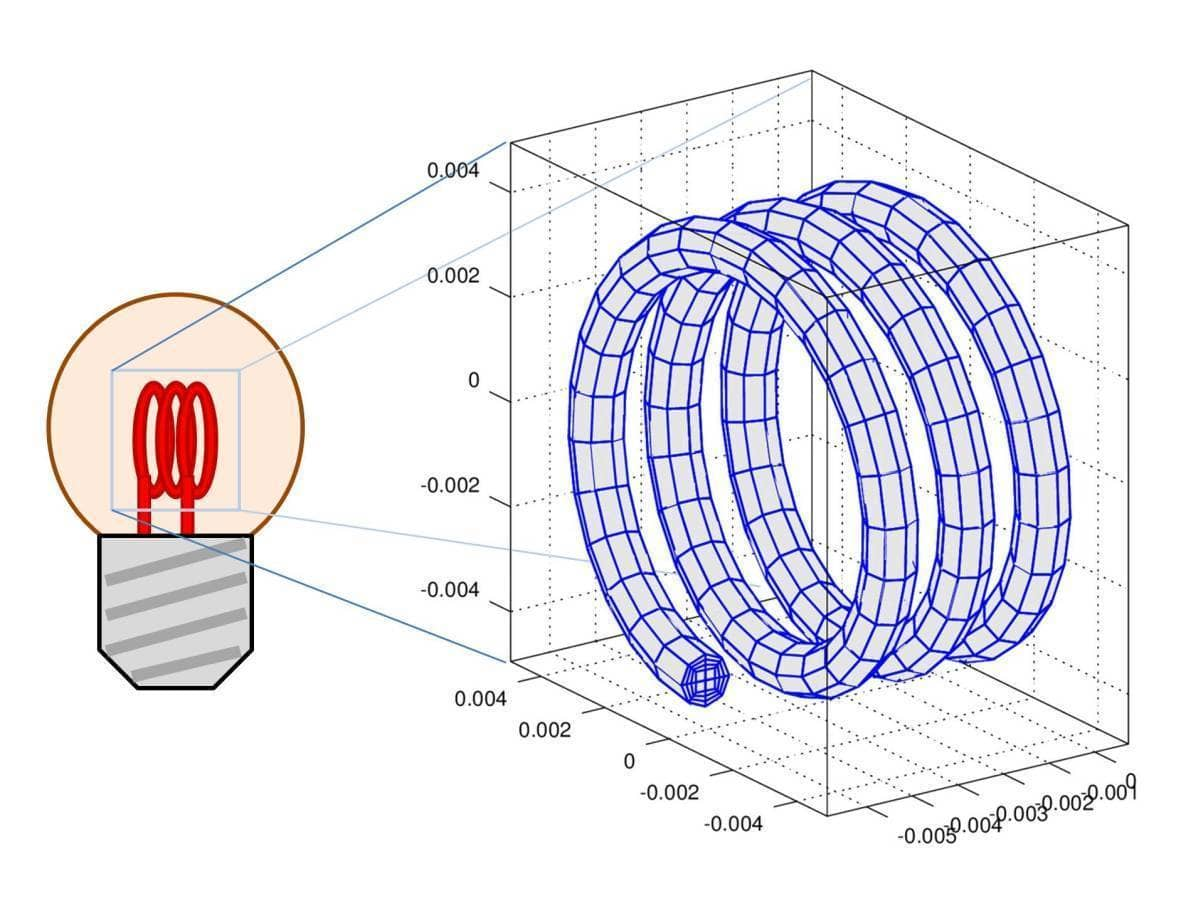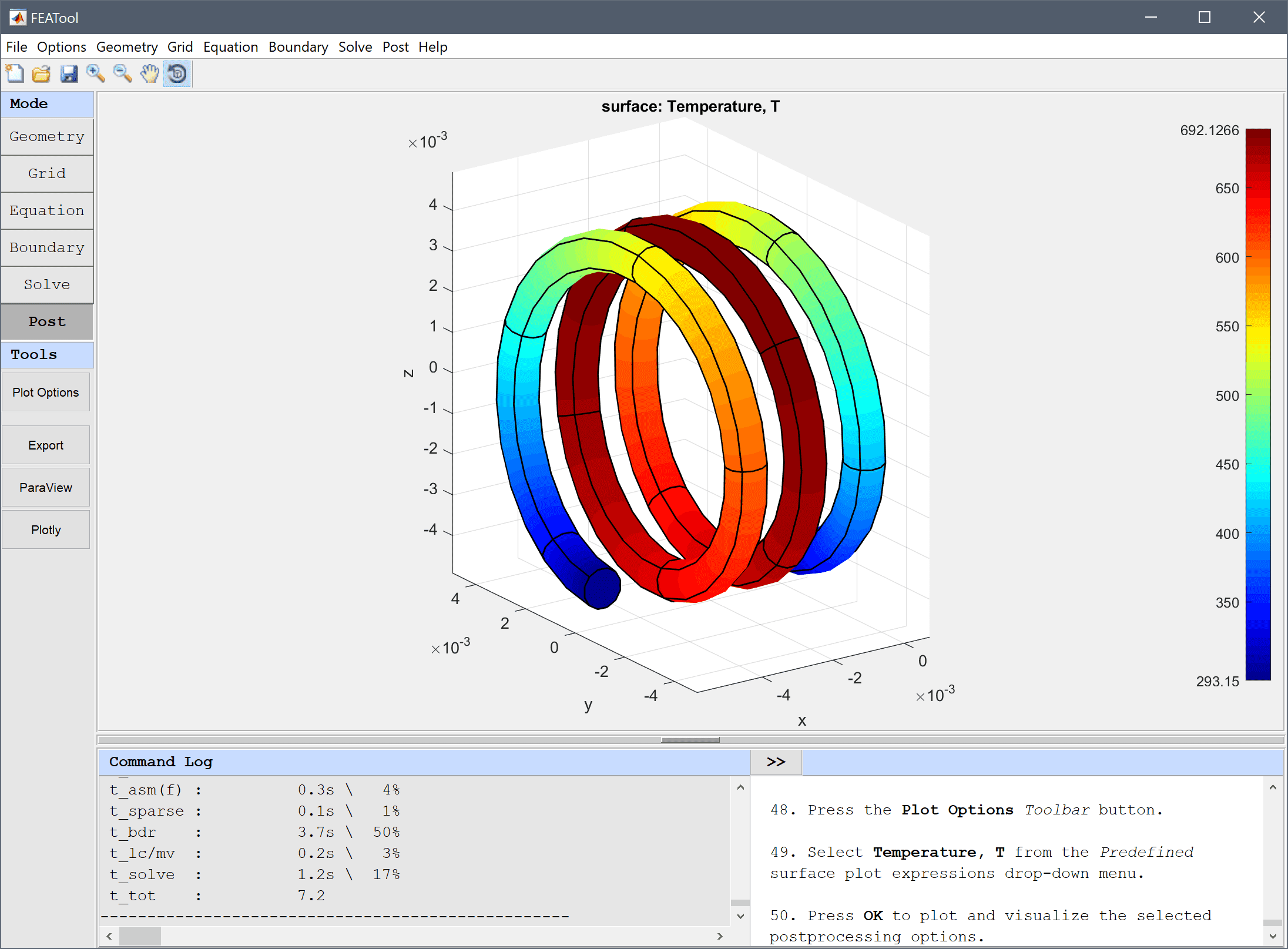# Resistive Heating in a Tungsten FilamentThis example models resistive Joule heating where the resulting current from an applied electric potential will heat a thin spiral shaped Tungsten wire, such as can be found in incandescent light bulbs. The filament reaches an equilibrium temperature where the internal heat generation is balanced by radiative heat loss through the boundaries.Two physics modes are involved, conductive media DC for the electric potential V, and heat transfer for the temperature T. Since the coupling is one way where only the heat transfer source term depends on the potential, the model will be solved in two steps. First for the electric potential, and then for the temperature using the pre-calculated potential, this saves computational time.

This model is available as an automated tutorial by selecting Model Examples and Tutorials… > Multiphysics > Resistive Heating in a Tungsten Filament from the File menu. Or alternatively, follow the linked step-by-step instructions.Tutorial Instructions

MATLAB Script Implementation